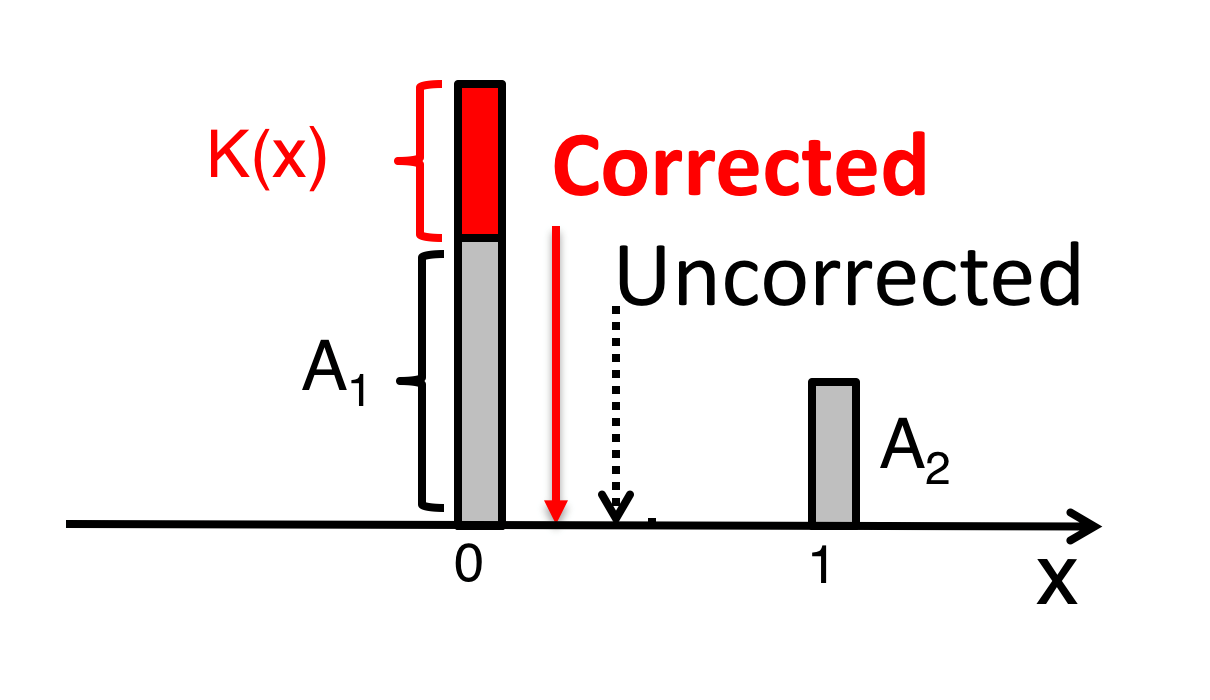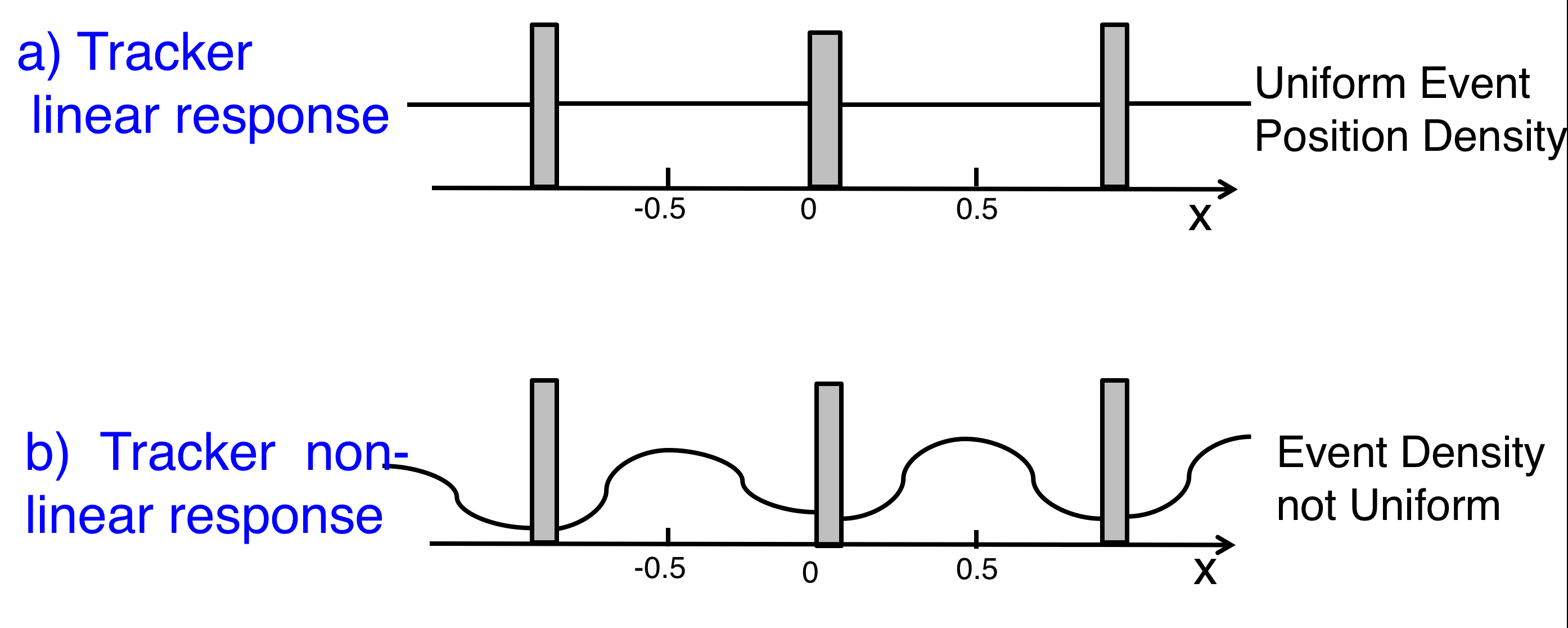# Tracker Coordinate Measurement Improvement in the Tracker Analysis

The y coordinate provides better accuracy by design, in which the readout strips have much smaller pitch compared to the strip pitch in the x coordinate. We present the improvement in the accuracy of determination of y-coordinate, which is the most important for the determination of momentum (or rigidity).

When a charged particle crosses a layer of the silicon tracker, its coordinate is determined by taking the ratio between the signals induced on the two strips between which the particle passed (see Figure 1).Figure 1. Schematic of particle coordinate measurement in the silicon tracker. The arrow indicates the point where the particle intersected the silicon sensor, the two adjacent strips are positioned at 0 and 1 in “strip” units. By definition, the maximum amplitude is defined as $A_{1}$ (at 0) and the next largest adjacent strip as $A_{2}$ (at 1). The AMS tracker has 196,608 strips. Traditionally, the ratio between amplitude A1 and A2 gives the particle coordinate, $x=1/(1+A_{1}/A_{2})$.

The amplitude of the induced signals should be proportional to $Z^2$. For $Z>3$ the amplitude $A_1$ starts to become non-linear, which causes resolution degradation. This effect is illustrated in Figure 2, where the spatial resolution for helium is compared with the resolutions for carbon and oxygen.Figure 2. Coordinate resolution for a) helium, b) carbon and c) oxygen events for the traditional analysis method.

We have developed an optimal way to correct for the non-linear effect. For this we need to identify a function $K(x$) which will restore the linearity of the amplitudes by taking into account amplitudes A1, A2 and the corrected position

$$x=1/(1+(A_{1}+K(x))/A_{2}) \tag{1}$$

This is illustrated in Figure 3.Figure 3. The principle of the optimal linearization: A1 is the non-linear amplitude and $K(x)$ the correction function. The dashed arrow shows the uncorrected coordinate measurement, the red arrow shows the corrected coordinate measurement.

To determine $K(x)$, we used the fact that cosmic rays are uniform and isotropic, so for an ideal tracker, without nonlinearity, we should see a uniform event position density (see Figure 4a), while in the non-linear case the event density is distorted (see Figure 4b).Figure 4. a) The uniform event position density for an ideal tracker. b) The distorted event position density for the tracker with non-linear amplitude response.

The function $K(x)$ was found to be different for different $|Z|$.  Figure 5 shows the event density distribution for carbon nuclei before and after correction.Figure 5. The event density before correction (black curve) and after correction (red curve). The strips are positioned at 0 for the strip with the amplitude $A_1$, and at 1 or -1 for $A_2$.

This method improved the position resolution by a factor of 2 for carbon (see Figure 6) and more than a factor of 2 for heavier nuclei.Figure 6. Comparison of the differences of the coordinates measured in tracker layers L3 or L5 to those obtained from the track fit using the measurements from L1, L2, L4, L6, L7, and L8, the residual, in the rigidity range $R > 50$ GV for a carbon sample using the traditional (old), as in Figure 11b, and new algorithms.

Figure 7 shows the coordinate accuracy for nuclei from $Z=2$ to $Z=26$ using the new analysis technique. Note that, due to the design of the tracker readout amplifier, the maximum non-linearity occurs for $Z\sim9$. This leads to the coordinate accuracy as a function of $Z$ shown in Figure 7.Figure 7. AMS tracker residuals σ as functions of nuclei charge Z for a) the L1-L9 geometry and b) the L1-L8 geometry.  The residuals were obtained by comparison of the differences of the coordinates measured in layers L3 or L5 to those obtained from the track fit using the measurements from L1, L2, L4, L6, L7, and L8 in the rigidity range $R > 50$ GV.